Next: 4.2 Modeling Approaches Up: 4. Mobility Modeling Previous: 4. Mobility Modeling

Subsections

# 4.1 Semiconductor Device Equations

The necessary equations which are solved in a numerical device simulator can be obtained from Maxwell's equations and Boltzmann's transport equation.

## 4.1.1 Maxwell's Equations

In differential and integral form, Maxwell's equations read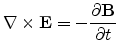Faraday's Law of InductionGauss's Law of Magnetism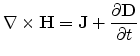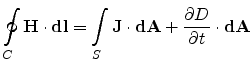Ampere's Circuital Law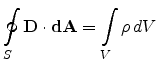Gauss's Law of Electrostatics

Here,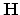denotes the magnetic field andthe magnetic flux density vector, while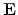corresponds to the electric field andto the electric displacement vector. They are related through the equations(4.1)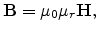(4.2)

where theand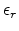denote the relative magnetic permeability and the relative dielectric permittivity of the medium, respectively.

The wavelength associated with an operating frequency of say,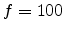GHz, is given by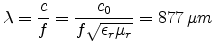(4.3)

Sinceis much greater than the typical device dimensions, which are of the order of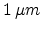, a quasi-stationary condition can be assumed for the electric field which can be expressed as a gradient of a scalar potential field,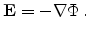(4.4)

Using (4.1) and (4.4) and Gauss's Law of Electrostatics, we obtain Poisson's equation,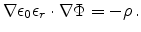(4.5)

The space charge density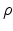in semiconductors comprises of the mobile charges and the fixed charges. Electrons and holes contribute to the mobile charges while fixed charges are the ionized donors and acceptors,(4.6)

Theanddenote the electron and hole concentrations and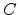corresponds to the net doping concentration.

Taking the divergence of Ampere's Circuital Law gives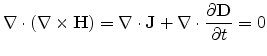(4.7)

The current densityin semiconductors is the sum of the electron and hole current densities denoted by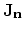and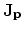.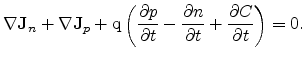(4.8)

Considering the fixed charges to be time-invariant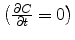, we get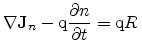(4.9)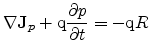(4.10)

The quantity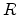gives the net recombination rate for electrons and holes. A positive value means recombination, a negative value generation of carriers. Equations (4.9) and (4.10) are collectively known as the carrier continuity equations.

## 4.1.2 Transport Equations and Mobility

Prediction of the I-V characteristics of semiconductor devices requires precise modeling of the mobility. A first principles calculation of mobility begins by describing the state of the electron gas in microscopic terms, followed by set of simplifying assumptions to arrive at macroscopic parameters that can describe the state of the gas collectively. A widely used approach for calculating mobility relies on the Boltzmann transport equation (BTE) which is an integro-differential equation based on both statistical and classical laws of dynamics.(4.11)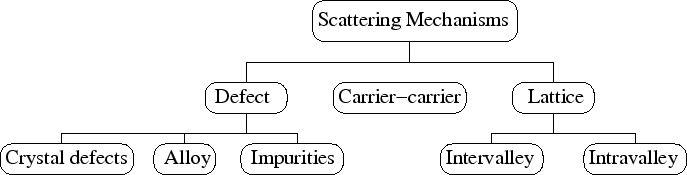Figure 4.1: Classification of different scattering mechanisms in Si adapted from [Lundstrom00].

Here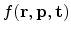denotes the single particle distribution function,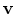denotes the group velocity of electrons andis the applied electric field. The left hand term in (4.11) describes the evolution of the distribution function with time in the six dimensional phase space of coordinates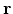and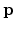in the presence of externally applied forces. The right hand side term corresponds to the effect of various scattering mechanisms on the distribution function.

Electrons and holes are accelerated by the electric field, but lose momentum as a result of various scattering processes. These scattering mechanisms contributing to the collision term in (4.11) are due to lattice vibrations (phonons), impurity ions, other carriers, surfaces, and other material imperfections. Fig. 4.1 shows a chart describing the various mechanisms of carrier scattering in a semiconductor. The effects of all of these microscopic phenomena are lumped into the macroscopic mobility introduced by the transport equation. However, in its original form, the BTE does not yield a closed form solution for mobility, and only after making certain assumptions, such as the relaxation time approximation, can the solutions be worked out. The resulting mobility can be expressed as(4.12)

where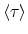denotes the average momentum relaxation time and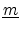is the effective mass tensor. The mobility expression in (4.12) is popularly referred to as the Drude model [Drude00]. It is apparent that the value ofdirectly affects the value of the mobility, and thus characterization of scattering mechanisms helps in estimating the mobility. For Si and Ge, the effective mass tensor is diagonal with equal diagonal components and therefore the mobility can be expressed as a scalar,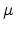.

In an actual sample of Si, multiple mechanisms can act to scatter the motion of electrons. Based on the assumption of the statistical independence of the scattering mechanisms, the scattering rates may be added using Matthiessen's rule [Nishida87]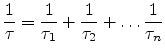(4.13)

whereindependent scattering mechanisms are involved. The overall mobility is therefore given by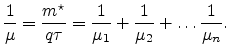(4.14)

## 4.1.3 Current Density

Transport in semiconductors can occur through the application of an electric field or through a gradient of carrier concentrations, temperature or material properties. Taking into consideration the effect of electric field and concentration gradients, an expression for the current density can be obtained from the Boltzmann's transport equation, as shown below.

Making use of the relaxation time approximation (RTA), the collision term on the RHS of (4.11) can be replaced by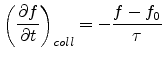(4.15)

where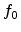denotes the equilibrium distribution function. Assuming steady state conditions, the one-dimensional BTE can now be written as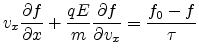(4.16)

Multiplying (4.16) with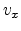and integrating over the three-dimensional velocity space gives(4.17)

Since the equilibrium function is symmetric, the first integral on the RHS in (4.17) vanishes, and the RHS of (4.17) becomes(4.18)

and therefore, we have,(4.19)

Evaluating the integrals in (4.19),(4.20)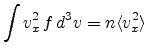(4.21)

Introducing the mobilityas in (4.12) and the average valueas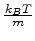, the current density in (4.19) becomes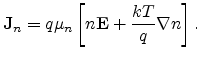(4.22)

A similar equation is obtained for the hole current density.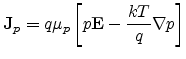(4.23)

The Poisson equation (4.5) together with the continuity equations (4.9) and (4.10) and the current density relations (4.22) and (4.23) constitute the fundamental equations for performing drift diffusion based simulations.

## 4.1.4 Basic MOS Equations

The drain current of an MOS transistor as a function of the gate and drain biases can be computed from the Poisson's equation by making the gradual channel approximation and the charge sheet approximation, as described in [Taur98].(4.24)

Here,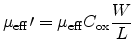, with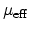as the carrier mobility andas the device width and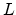as the gate length. In the linear regime, where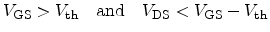(4.25)

the drain current expression can be simplified to(4.26)

In the saturation regime, where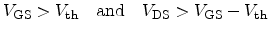(4.27)

the drain current is modeled as(4.28)

wheredenotes the channel length modulation parameter. The quantitydenotes the threshold voltage and is obtained as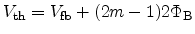(4.29)

Here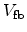is the flat band voltage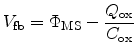(4.30)

and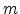is the body-effect coefficient defined as(4.31)

The potentialis evaluated as(4.32)

In (4.31),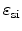is the permittivity of the Si substrate,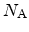the acceptor doping concentration, andthe capacitance per unit area of the oxide. The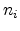denotes the intrinsic carrier concentration of Si,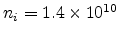cm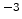at 300 K.

From equations (4.26) and (4.28) it is seen that the drain current is directly proportional to the mobility. Therefore, employment of strained Si, which enhances the mobility, results in an increase in the drain current, thereby making circuits faster. Moreover, since strain causes a relative shift of the conduction and valence band minima, it can result in a reduced threshold voltage due to a decreased work function difference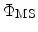.Next: 4.2 Modeling Approaches Up: 4. Mobility Modeling Previous: 4. Mobility Modeling

S. Dhar: Analytical Mobility Modeling for Strained Silicon-Based Devices